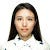Data Types:

• float: Numbers with a decimal part, even if that part is zero ( eg 2.5,3.141,-3.0)
• str: Strings are how we store text data in Python. Strings are strings of characters between either double quotes (“”) or single quotes (‘’)

Classification Metrics — Study Notes

Accuracy=(TP+TN)/All Predictions

Misclassification Rate=(FP+FN)/All Predictions or 1-Accuracy

Sensitivity=TP/(TP+FN)

• a.k.a. True Positive Rate, Recall

Specificity=TN/(TN+FP)

• a.k.a. True Negative Rate

Precision=TP/(TP+FP)

• a.k.a. Positive Predictive Valu

F1 score=2*(Precision*Recall)/(Precision+Recall)

• F1 score is the harmonic mean of precision and recall, if you care about precision and recall roughly the same amount, F1 score…

Regression Metrics — Study Notes

Residuals- we can plot residuals out and look for Scedasticity and Outliers, this is how a Homoscedasticity residuals plot look like:

Mean Absolute Error (MAE): goal is to get mae as close to 0 as possible:

• Pros: Represents median distance from the predicted value; In the original units of Y…

Linear Regression Model-Study Notes

Ordinary Least Squares Regression: linear regression that has minimum mean squared error(MSE).

Multiple Linear Regression: when we have more than 1 predictor variable

• When we interpret the coefficients from Multiple Linear Regression, we have to say ‘when all else hold’ before interpret any one of the coefficients.

Assumptions for Linear…

There are 3 types of errors that a models can have, error due to bias, error due to variance, and irreducible errors due to randomness or natural variability in an existing system. Linear regression model is usually low variance and high bias.

Bias:

• Underfitting